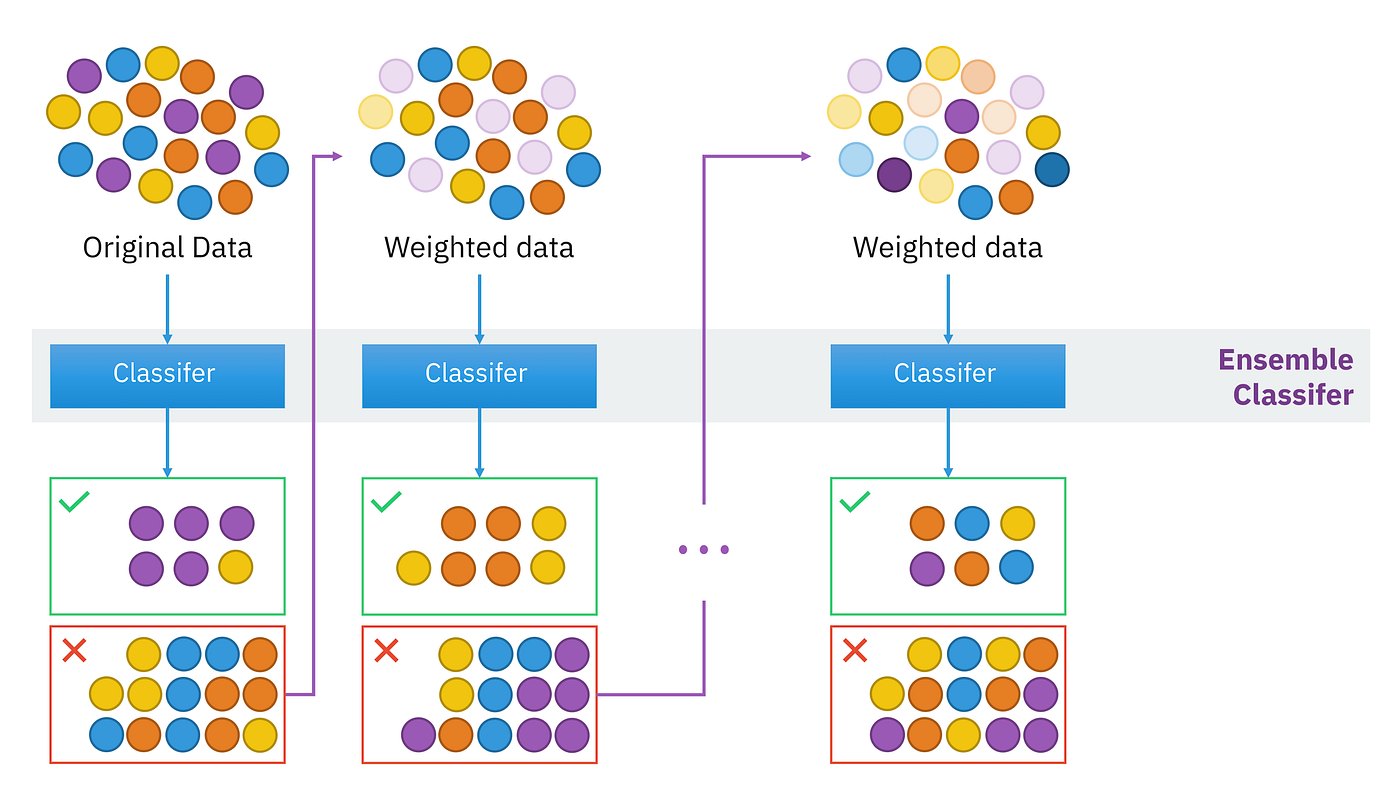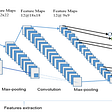# A Deep Dive into XGBoost

eXtreme Gradient Boosting (XGBoost) is a highly scalable end-to-end tree boosting system developed by Tianqi Chen and Carlos Guestrin at the University of Washington. They introduced several optimization techniques, which we will study in-depth, that achieved state-of-the-art results on many machine learning problems.

Since its introduction, it has been widely recognized and has dominated several machine learning challenges in Kaggle and other competitions. Before we dive into XGBoost, let's talk about Boosting.

## Boosting

Boosting is a sequential ensemble learning technique that uses several weak learners to develop a strong performing model. It combines prediction through a weighted majority vote or a weighted sum from several models to produce the final prediction. Rather than training different models separately, boosting trains them in succession and the new model is trained to correct the error made by the previous one. It will adjust the weight of an observation based on the last prediction by increasing its weight if incorrectly predicted and vice-versa. The model that performs better will have a higher weight in the final ensemble model.

This way it will convert several weak learners into a strong one and can be further tuned (as we will learn later) based on the data to increase accuracy.Illustration of a boosting method for ensemble learning. source: Sirakorn via Wikipedia

# Why XGBoost is so effective?

Now that we know how boosting works, let's look into some of the improvements made in the XGBoost paper.

As stated in the paper:

“The most important factor behind the success of XGBoost is its scalability in all scenarios. The system runs more than ten times faster than existing popular solutions on a single machine and scales to billions of examples in distributed or memory-limited settings. The scalability of XGBoost is due to several important systems and algorithmic optimizations. These innovations include: a novel tree learning algorithm is for handling sparse data; a theoretically justified weighted quantile sketch procedure enables handling instance weights in approximate tree learning. Parallel and distributed computing make learning faster which enables quicker model exploration.”

## Algorithmic Optimization:

• Regularized Learning Objective: The objective function is defined to measure how well the model fits the training data. It consists of two parts: training loss and a regularization term. The regularization term penalizes the complexity of the model and helps to smooth the final learned weights to avoid over-fitting. So, the regularized objective will tend to select a model employing simple and predictive functions.

Here, Ω term penalizes the complexity of the model.

• Shrinkage and Column Subsampling: Shrinkage scales newly added weights by a factor η after each step of tree boosting and reduces the influence of each tree and leaves space for future trees to improve the model. Column sub-sampling helps to prevent over-fitting while also speeding up computations of the parallel algorithm implemented in the model.
• Split-finding Algorithms: One of the major problems in tree learning is finding the best split. To do so, the exact greedy algorithm enumerates all the possible splits on all the features. It is very powerful since it looks over all the possible splitting points greedily. However, the possible splits for continuous features can be very large and it is computationally demanding to enumerate over all the splits and an approximate algorithm is introduced to address this. The algorithm first proposes candidate splitting points according to percentiles of feature distribution then maps the continuous features into buckets split by these candidate points, aggregates the statistics, and finds the best solution based on the aggregated statistics.
• Weighted Quantile Sketch: To effectively find the optimal split points among weighted datasets, a novel distributed weighted quantile sketch algorithm was introduced that can handle weighted data with a provable theoretical guarantee.
• Sparsity-aware Split Finding: It is common for input to be sparse due to missing values in the data, a high number of zero entries, or after performing feature engineering such as one-hot encoding. To make the algorithm aware of the sparsity patterns in the data, XGBoost adds a default direction in each tree node. Depending on the feature, a missing value will direct the decision along the left or right path and will handle all sparsity patterns in a unified way. The key point is to only visit the non-missing entries which made the algorithm 50x faster than the naïve approach.

## System Improvements:

• Column Block for Parallel Learning: In tree learning, sorting the data is very time-consuming and to reduce this cost, the paper proposed to store the data in in-memory units called block. In each block, data is stored in the compressed column format, with each column sorted by the corresponding feature value. XGBoost sorts each block parallelly utilizing all available threads of CPU.
• Cache-aware Access: By using the cache-aware method, an internal buffer is allocated in each thread and gradient statistics are fetched into it to perform accumulation in a mini-batch manner. This reduces the runtime overhead when the number of rows in the dataset is large. Cache-aware implementation of the exact greedy algorithm runs twice as fast as the naïve version with a large dataset and is achieved by choosing the optimal size of the block (found to be ²¹⁶).
• Blocks for Out-of-core Computation: Techniques such as Block Compression and Block Sharding are used to utilize disk space to handle data that does not fit into main memory. To enable out-of-core computation, data is divided into multiple blocks and each block is stored on disk. In Block Compression, the block is compressed by columns, and decompressed on the fly by an independent thread when loading into main memory. Block Sharding shares the data onto multiple disks in an alternative manner.

# Hyperparameters Tuning

One of the most important tasks in Machine Learning is to properly tune the hyperparameters. XGBoost has large sets of parameters that can be tuned and it might be difficult to get your head around. Let’s look into some of the hyperparameters that are important to understand when creating an XGBoost model.

• max_depth, range: [0, infinity]: It is the maximum depth of the tree and controls the complexity of the model. Higher the number of max_depth, the higher the chance of overfitting so it is recommended to start with low max_depth (such as 4).
• eta or learning_rate, range: [0,1]: It is the step size shrinkage used in the update to prevent overfitting. Learning Rate shrinks the feature weights to make the model more robust and the boosting process more conservative.
• min_child_weight, range: [0, infinity]: It is the minimum sum of instance weight (hessian) needed in a child and defines how big each group in the tree has to be. The larger min_child_weight is, the more conservative the algorithm will be. The higher the max_depth is, the higher min_child_weight should be to prevent overfitting.
• colsample_bytree, range: (0, 1]: It is the fraction of columns to be randomly sampled for each tree. The value for this depends on your data and the number of features present.
• gamma or min_split_loss, range: [0, infinity]: Mathematically known as the “Lagrangian multiplier”, gamma is the minimum loss reduction required to make a further partition on a leaf node of the tree. The value for the optimal gamma depends on the training dataset and other parameters used in the model. The larger the gamma is, the higher the regularization is and the more conservative the algorithm will be. In gamma, we will prune a shallow tree using the loss function instead of hessian weight (in min_child_weight). It can push the model beyond the local performing maxima and even a small difference in its value will affect the model.
• lambda [default=1] and alpha [default=0]: Lambda is L2 regularization (analogous to Ridge Regression) and Alpha is L1 regression (analogous to Lasso Regression). Increasing the value of these will make a model more conservative.
• subsample, range: (0,1]: It is the fraction of observations to be randomly sampled for each tree. The subsample value of 0.6 means that the model will randomly sample 60 percent of the training data prior to growing its trees. Lower values will make a model more conservative and prevent overfitting but values too small might lead to under-fitting.

Note: Methods such as Hyperopt, Grid search and Random Search can be used to tune these hyperparameters to find the optimal values.

# Example

Let’s create a simple XGBoost Regression model for the Boston Housing dataset:

And to plot the feature importance, we can just run: xgb.plot_importance(gbm)

# Conclusion

Hopefully, this has provided you with a general understanding of how XGBoost works and why it is so effective. I highly recommend reading the original paper and getting your hands dirty by implementing it yourself.

Happy coding!

# Reference

--

--

--

## More from Manoj Aryal

software engineer

Love podcasts or audiobooks? Learn on the go with our new app.

## SentiCart: Cartography and Geo-contextualization for Multilingual Visual Sentiment## Object detection with Raspberry Pi and Python## Zero-Shot Learning## The emergence of A.I. for text generation## Word Embeddings## 5 questions to the core developer## Introduction to Convolutional Neural Networks — Deep Learning## Manoj Aryal

software engineer

## What is Machine Learning## Accuracy is not accurate.## Understanding the Backpropagation Algorithm